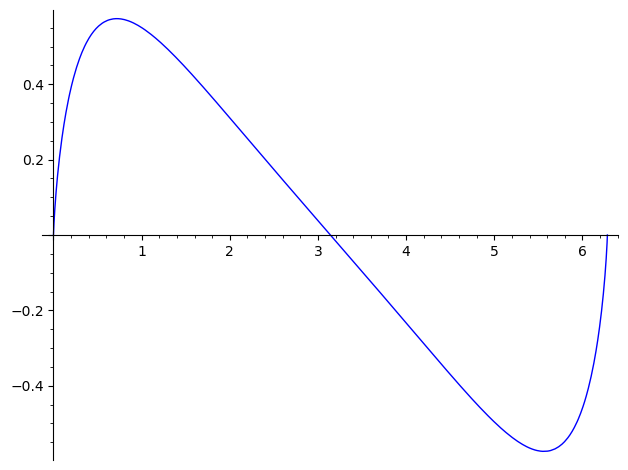Ask Your Question

# limit of fourier seriesI'd like to compute the limit of $$\sum_{k=1}^{n}\frac{1}{k^2+1}\sin(kx)$$ I did the following already:

def b(k):
return(1/(k**2+1))
def a(k,x):
return(b(k)*sin(k*x))
def s(n,x):
s=0
for k in range(1,n+1):
s=s+a(k,x)
return(s)
var('x,n')
f=s(1000,x)
plot(f,0,2*pi)


I already tried computing it by hand, looked in Bronstein, searched the internet, but didn't find any solution. But I'm no specialist in Analysis, so perhaps somebody can help? Clearly the series converges for every x in [0,2*pi] ...

edit retag close merge delete

## 1 Answer

Sort by » oldest newest most votedHere is what it looks like

sage: def f(x):
....:     return sum(sin(k*x)/(k**2+1) for k in range(1000))
sage: plot(f,0,2*pi)


Not clear if this is a known function.more

## Comments

Indeed, and the error is bounded by:

sage: var('k')
k
sage: sum(1/(k^2+1),k,1001,oo).n()
0.000999499833833166


Yes, but it does not answer my question ;-) I want to compute the limit function f(x), restricted to x from 0 to 2*\pi

## Your Answer

Please start posting anonymously - your entry will be published after you log in or create a new account.

Add Answer

## Stats

Asked: 2021-02-06 10:00:40 +0200

Seen: 67 times

Last updated: Feb 07